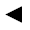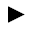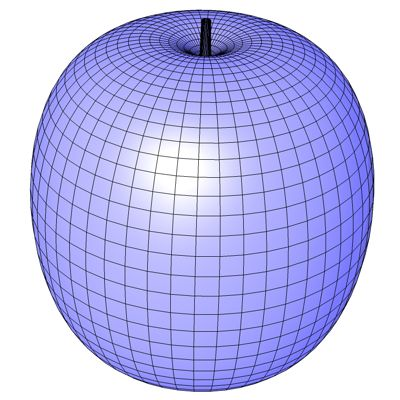﻿﻿﻿ math handbook calculator - Fractional Calculus Computer Algebra System software+++=﻿[back]

## 3.50. Apple Surface

[next]The Apple Surface [ 8 ] is represented by the following equations.

 x = cos(u) (4 + 3.8 cos(v)) 3-165 y = sin(u) (4 + 3.8 cos(v)) 3-166 z = (cos(v)+sin(v)-1) (1+sin(v)) log(1-pi v/10)+7.5 sin(v) 3-167

To represent the area, the two parameters u and v can assume the following values ​​(domain of definition), for example.

 u is an element from the set of numbers [0, 2 pi] v is an element from the number set [-pi, pi]

The domain of definition cannot be changed in the plugin.
The plugin creates an optimized mesh without duplicate points and unconnected polygons.Fig. 54